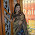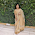Dear students,

Numerical Ability for SBI Clerk Prelims 2018

Today is the Day 53 of the SBI Clerk 60 Days Study PlanNumerical Ability Section has given heebie-jeebies to the aspirants when they appear for a banking examination. As the level of every other section is only getting complex and convoluted, there is no doubt that this section, too, makes your blood run cold. The questions asked in this section are calculative and very time-consuming. But once dealt with proper strategy, speed, and accuracy, this section can get you the maximum marks in the examination. Following is the Numerical Ability quiz to help you practice with the best of latest pattern questions.

Q1. A man goes to a place on bicycle at speed of 16 kmph and comes back with another speed. If the average speed of the whole journey is 6.4 kmph, then find the speed of return journey.
(a)4 kmph
(b)6 kmph
(c)7 kmph
(d)7.5 kmph
(e)3 kmph

Q2. The average of one hundred observations was calculated as 35. It was found later, that one of the observation was read as 83 instead of 53. What is the correct average?
(a)33.7
(b)34.2
(c)34.7
(d)34.8
(e)None of these

Q3. Two barrels contain a mixture of ethanol and gasoline. The content of ethanol is 60% in the first barrel and 30% in the second barrel. In what ratio must the mixtures from the first and the second barrels be taken to form a mixture so that new mixture contain 50% ethanol?
(a)1 : 2
(b)2 : 1
(c)3 : 2
(d)5 : 3
(e) 7: 5

Q4. The ratio of two numbers is 3/2:8/3. If each of the number is increased by 15 the ratio becomes 5/3:5/2. Find the bigger number.
(a) 27
(b) 36
(c) 48
(d) 64
(e) 72

Q5. First type of liquid contains 25% of milk, the other second type of liquid contains 30% of milk. A container is filled with 6 liters of the first liquid and 4 liters of the second liquid. The percentage of milk in the mixture is:
(a) 27%
(b) 31%
(c) 29%
(d) 33%
(e) 37%

Solutions (1-5):

Q6. A, B, and C have amounts in the ratio of 3 : 4 : 5. First B gives 1/4 th to A and 1/4 th to C then C gives 1/6 th to A. Find the final ratio of amount of A, B and C, respectively:
(a) 4 : 3 : 5
(b) 5 : 4 : 3
(c) 6 : 4 : 2
(d) 5 : 2 : 5
(e) 7: 3: 5

Q7. Average of marks obtained by Ram in 4 different subjects was 75. But later it was found that marks of two subjects were written incorrectly as 69 and 86 in place of 96 and 68 respectively. Find the correct average of marks?
(a) 85.50
(b) 82
(c) 77.25
(d) 72.25
(e) 73.75

Q8. Amit spends 75% of his income. If his income was increased by 20% and he increased his expenditure by 10%, by how much percent his saving increased?
(a) 25%
(b) 30%
(c) 35%
(d) 50%
(e) 60%

Q9. A sum was distributed among A, B, C in the ratio of 2 : 5 : 9. If part of A was 2500 rupee, what is the total sum?
(a) Rs. 20000
(b) Rs. 25000
(c) Rs. 22000
(d) Rs. 15000
(e) Rs. 18000

Q10. A, B and C start a business together by investing a total amount of Rs. 50000. Sum of A is Rs. 4000 more than that of B and sum of B is Rs. 5000 more than that of C. If total profit at the end of a year was found to be Rs. 35000, what is the share of A in the profit?
(a) Rs. 11900
(b) Rs. 14700
(c) Rs. 13600
(d) Rs. 8470
(e) None of these

Solutions (6-10):

Q11. Three persons A, B and C enter into a business with their initial capital of Rs. 15,000. Rs. 12000 and Rs. 18000 respectively. After 6 months, C withdrew 6000 and B invests an additional amount of 3000. After a year total profit is Rs. 6,670. Find what is the share of B in the profit?
(a) Rs. 2,250
(b) Rs. 2,007
(c) Rs. 2,150
(d) Rs. 2,070
(e) None of these

Q12. If the numerator of a fraction is increased by 20% and denominator is reduced by 30%, the new fraction thus formed is 8/21. Find the original fraction
(a) 2/9
(b) 4/9
(c) 5/9
(d) 9/2
(e) None of these

Q13. The average of seven numbers is 21. If the average of first three numbers is 19 and that of last three numbers is 20, what is the fourth number from starting?
(a) 35
(b) 32
(c) 30
(d) 40
(e) 41

Q14. Two persons X and Y enter into a business by investing their sum in the ratio of 5 : 6. After 8 months, X leaves the business. After a year, if total profit is Rs. 1260, what is the profit of X?
(a) Rs.445
(b) Rs.450
(c) Rs.440
(d) Rs.460
(e) Rs.480

Q15. The average age of 32 persons is 20 years. Due to entry of 2 more persons, the average age increases by 1 year. Find the average age of two new persons.
(a) 35 years
(b) 34 years
(c) 37 years
(d) 33 years
(e) 22 years1.question no 6's answer don't understand

2.question no 6's answer don't understand

3.plz explain ques no 1 in detail

4.formula for average speed is
= 2(s1*s2)/s1+s2
now let the speed of return journey is s2
2*16*s2/16+s2= 6.4
s2=4kmph

5.there is nothing to do in que.6. only u have to calculate the ratios. calculate by letting the no.s as 18:24:30 now it will be convinient for u to solve

6.This comment has been removed by the author.